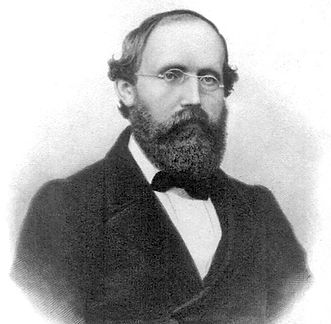# More on the Recursive RelationshipHere the relation between the sums is explained. The real and imaginary sums are the differences of the even and odd sums, while the sine and cosine sums from the even portions are the sums of the even and odd sums. Due to the original requirement that both the real and imaginary sums must be equal to 0, and due to the discovered relationships between the sums, new conditions and relationships can be stated for the sums.

*Note, the statement "Adding 2 copies of the corresponding odd function to each side of equation 32..." could be misinterpreted as written to people unfamiliar with the process, as was pointed out by someone doing peer review. Technically, it means the right sides and middle portions as equation 32 is stated, since 32 states the equivalence of 3 equations for each portion. The A and B in 32 were carried along for reference, but not really required as part of that statement. Someone familiar with the process would know what was intended, but someone less familiar could be lead astray. Another way to say it, is to just use eq 33 with eq 36 and 37, to get eq.38.